# Kruskal’s Minimum Spanning Tree Algorithm

The Kruskal’s Minimum Spanning Tree Algorithm is an algorithm which is used to construct a Minimum Spanning Tree for a connected weighted graph. Kruskal’s algorithm to find the minimum cost spanning tree uses the greedy approach. This algorithm works on principle of finding the subset of the edges from the given graph and covering each and every vertex in a graph such that tree is formed and sum of weights of these edges minimum possible.

In this greedy algorithm, a Greedy Choice is made by putting the smallest weight edge until all vertex are covered and does not violate MST properties.

## Example of Kruskal’s Algorithm

Greedy algorithm approach is used to find the minimum cost spanning tree. Using this appraoch, Greedy Choice is made by putting the smallest weight edge.

Below is the example of Kruskal’s Algorithm with input- output constraint and the solution for the example.

### Input and Output of the Example

Given the undirected, connected and weighted graph G, and the task is used to find the minimum cost spanning tree.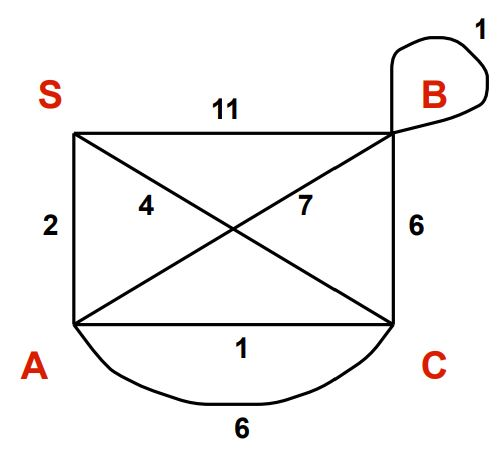Solution: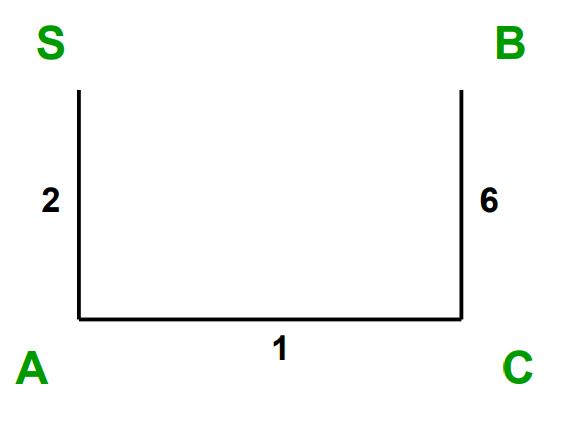### Steps for finding MST using Kruskal’s Algorithm

Step 1: Remove all loops and Parallel Edges with larger weights.

Step 2: Sort all the edges in non-decreasing order of their weights.

Step 3: Add the edge which has the least weightage. Check if minimum spanning tree property follows then include this edge.

Step 4: Repeat Step 2 until all vertices are covered.

### Solution of the Problem Example

1. Given the undirected, connected and weighted graph G.2. Remove all loops and Parallel Edges with larger weights.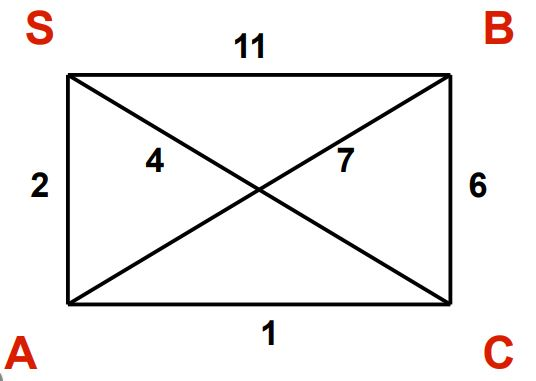3. After sorting edge of G in order of increasing weight.

Weight Edge
1 A <–> C
2 S <–> A
4 S <–> C
6 B <–> C
7 A <–> B
11 S <–> B
4. Picking all edges from sorted list of edges and Addition of the edge which has the smallest weight.

• The least weight is 1 and pick the edge A <–> C, since the MST property is follow add it to the solution.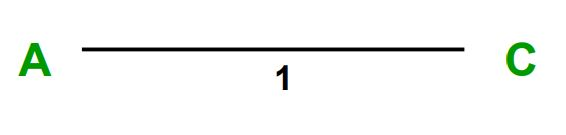• Next smallest weight is 2, pick the edge S <–> A, since the MST property is follow add it to the solution.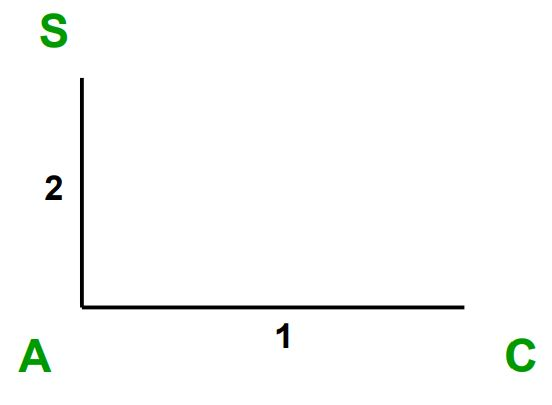• Next smallest weight is 4, pick the edge S <–> C, here the MST property is not follow and cycle is formed. No update to the solution.
• Next smallest weight is 6, pick the edge B <–> C, since the MST property is follow add it to the solution.• Next smallest weight is 7, pick the edge A <–> B, here the MST property is not follow and cycle is formed. No update to the solution.
• Next smallest weight is 11, pick the edge S <–> B, here the MST property is not follow and cycle is formed. No update to the solution.
1. Minimum Cost Spanning Tree is :## Pseudocode for Kruskal’s algorithm

Here’s pseudocode to find the minimum cost spanning tree in a graph using kruskal’s algorithm (greedy algorithm approach).

``````Kruskal_algorithm(Graph)

ANS <- ∅

for each Vertex v ∈ Graph.Vertex
do
Make_SET(v)

for each Edge (u, v) in Graph.Edge for increasing order of weight(u, v)
do
if Find_in_SET(u) ≠ Find_in_SET(v)
do
ANS := ANS ∪ {(u, v)}
UNION(Find_in_SET(u), Find_in_SET(v))

return ANS
``````

## Implementation of Kruskal’s Algorithm in Python

``````# Kruskal's algorithm in Python

# Function to print the soluton
def print_sol():
global Solution
for vertex_1, vertex_2, weight in Solution:
print("(%d , %d) -> %d" % (vertex_1, vertex_2, weight))

# function to add vertex pair with weight
def add_edge(vertex_1, vertex_2, weight):
global Graph
Graph.append([vertex_1, vertex_2, weight])

# Search function
def search_for( res_set1, i):
if res_set1[i] == i:
return i
return search_for(res_set1, res_set1[i])

# Function to do union of two sets
def union_set( res_set1, res_set2, Set_1, Set_2):

x = search_for(res_set1, Set_1)
y = search_for(res_set1, Set_2)

# smaller rank tree under root of
# high rank tree
if res_set2[x] > res_set2[y]:
res_set1[y] = x

elif res_set2[x] < res_set2[y]:
res_set1[x] = y

else:
res_set1[y] = x
res_set2[x] += 1

# Function to illustrate the Kruskal algorithm
def Kruskal_algorithm():

global Solution, Graph

# Variable INX used for sorted edges
# Variable NXT used for Soltion
INX, NXT = 0, 0

#Sort Graph in non-decreasing order of weight
Graph = sorted(Graph, key=lambda item: item)

res_set1 = []
res_set2 = []

for node in range(V):
res_set1.append(node)
res_set2.append(0)

while V - 1 > NXT:

vertex_1, vertex_2, weight = Graph[INX]
INX = INX + 1

Set_1 = search_for(res_set1, vertex_1)
Set_2 = search_for(res_set1, vertex_2)

if Set_1 != Set_2:

union_set(res_set1, res_set2, Set_1, Set_2)
Solution.append([vertex_1, vertex_2, weight])
NXT = NXT + 1

# Driver Program

# Number of vertices
V = 4

# To store the Graph
Graph = []

# Adding the weight with vetices
add_edge(0, 1, 2)
add_edge(0, 2, 4)
add_edge(0, 3, 11)
add_edge(1, 0, 2)
add_edge(1, 2, 1)
add_edge(1, 3, 7)
add_edge(1, 2, 6)
add_edge(2, 0, 4)
add_edge(2, 1, 1)
add_edge(2, 1, 6)
add_edge(2, 3, 6)
add_edge(3, 0, 11)
add_edge(3, 1, 7)
add_edge(3, 2, 6)
add_edge(3, 3, 1)

# To store the resultant MST
Solution = []

# Function calling
Kruskal_algorithm()

# Printing the Solution
print_sol()
``````
Scroll to Top

### Full Stack Integrated Bootcamp Free Trial

• Please enter a number from 7000000000 to 9999999999.